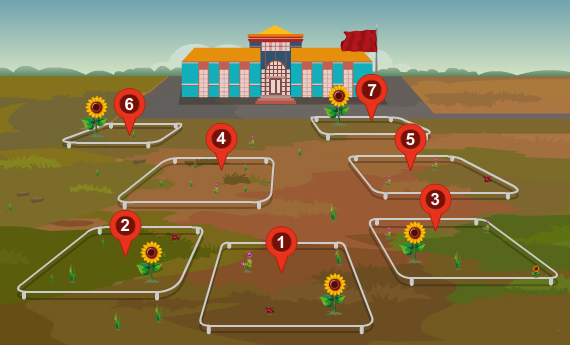Study of plant population density by quadrat method

# Aim

Our aim is to study plant population density by the quadrat method.

# Theory

A population is a group of individuals of the same species which inhabit a particular space at a particular time. The number of individuals in a population never remains constant. It may increase or decrease due to many factors like birth rate, death rate and migration. The number of individuals of the species in any unit area at a given time is its population density. The unit area may be as small as 5 square centimeters to as large as 10 square metres, depending on the size and nature of the plant community under study.

## Let’s understand the Quadrat Method.

Counting all individuals in a population is the most accurate way to determine its size. However, this approach is not usually feasible, especially for large populations or extensive habitats. Scientists usually calculate plant populations with the quadrat method. A quadrat is a square that encloses an area within a habitat.  For herbaceous vegetation, a metre square quadrat is normally used.Once analyzed, the sample data enables the scientist to calculate the population size and population density for the entire population. Population density is calculated by counting all the individuals present at a given time in a given space, divided by the number of units of area or space.

### Population density is calculated as follows:

Density   = (Total no.of individuals of the species in all the sampling unit (S))/(Total number of sampling units studied (Q))

# Learning Outcomes:

• Students understand the term quadrat method.
• Students understand how to calculate plant population density.
• Students do the experiment better in the real lab having gone through the animation and simulation.

Cite this Simulator: